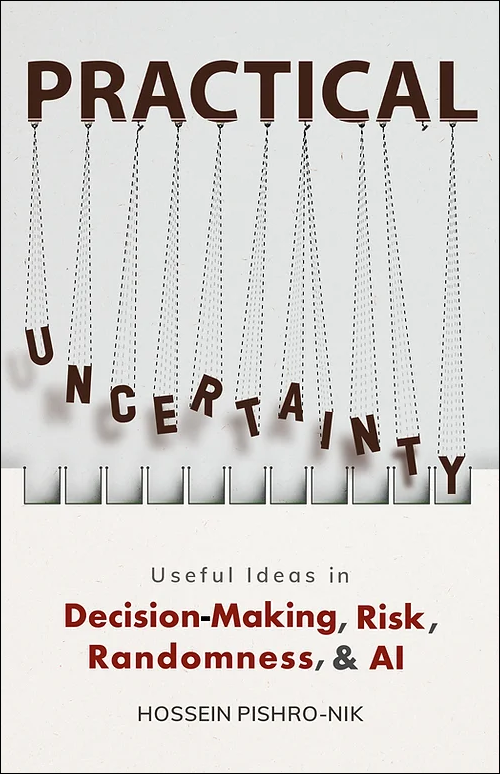## 6.2.3 Chernoff Bounds

If $X$ is a random variable, then for any $a \in \mathbb{R}$, we can write \begin{align}%\label{} P(X \geq a)= P(e^{sX} \geq e^{sa}), &\qquad \textrm{ for } s>0, \\ P(X \leq a)= P(e^{sX} \geq e^{sa}), &\qquad \textrm{ for } s<0. \end{align} Now, note that $e^{sX}$ is always a positive random variable for all $s \in \mathbb{R}$. Thus, we can apply Markov's inequality. So for $s>0$, we can write \begin{align}%\label{} P(X \geq a)&= P(e^{sX} \geq e^{sa})\\ & \leq \frac{E[e^{sX}]}{e^{sa}}, \hspace{10pt} \textrm{ by Markov's inequality}. \end{align} Similarly, for $s<0$, we can write \begin{align}%\label{} P(X \leq a)&= P(e^{sX} \geq e^{sa})\\ & \leq \frac{E[e^{sX}]}{e^{sa}}. \end{align} Note that $E[e^{sX}]$ is in fact the moment generating function, $M_X(s)$. Thus, we conclude

Chernoff Bounds:

 $P(X \geq a)\leq e^{-sa}M_X(s),$ $\textrm{ for all }s>0$, $P(X \leq a)\leq e^{-sa}M_X(s),$ $\textrm{ for all }s<0$

Since Chernoff bounds are valid for all values of $s>0$ and $s<0$, we can choose $s$ in a way to obtain the best bound, that is we can write \begin{align}%\label{} P(X \geq a)& \leq \min_{s>0} e^{-sa}M_X(s), \\ P(X \leq a)&\leq \min_{s<0} e^{-sa}M_X(s). \end{align} Let us look at an example to see how we can use Chernoff bounds.

Example

Let $X \sim Binomial(n,p)$. Using Chernoff bounds, find an upper bound on $P(X \geq \alpha n)$, where $p< \alpha<1$. Evaluate the bound for $p=\frac{1}{2}$ and $\alpha=\frac{3}{4}$.

• Solution
• For $X \sim Binomial(n,p)$, we have \begin{align}%\label{} M_X(s)=(pe^s+q)^n, &\qquad \textrm{ where }q=1-p. \end{align} Thus, the Chernoff bound for $P(X \geq a)$ can be written as \begin{align}\label{eq:cher-1} P(X \geq \alpha n)& \leq \min_{s>0} e^{-sa}M_X(s)\\ \ &= \min_{s>0} e^{-sa}(pe^s+q)^n. \end{align} To find the minimizing value of $s$, we can write \begin{align}%\label{} \frac{d}{ds} e^{-sa}(pe^s+q)^n=0, \end{align} which results in \begin{align}%\label{} e^{s}=\frac{aq}{np(1-\alpha)}. \end{align} By using this value of $s$ in Equation 6.3 and some algebra, we obtain \begin{align}%\label{} P(X \geq \alpha n)& \leq \big( \frac{1-p}{1-\alpha}\big)^{(1-\alpha)n} \big(\frac{p}{\alpha}\big)^{\alpha n}. \end{align} For $p=\frac{1}{2}$ and $\alpha=\frac{3}{4}$, we obtain \begin{align}%\label{} P(X \geq \frac{3}{4} n)& \leq \big(\frac{16}{27}\big)^{\frac{n}{4}}. \end{align}

Comparison between Markov, Chebyshev, and Chernoff Bounds:

Above, we found upper bounds on $P(X \geq \alpha n)$ for $X \sim Binomial(n,p)$. It is interesting to compare them. Here are the results that we obtain for $p=\frac{1}{4}$ and $\alpha=\frac{3}{4}$: \begin{align}%\label{} &P(X \geq \frac{3n}{4})\leq \frac{2}{3} \hspace{58pt} \textrm{Markov}, \\ &P(X \geq \frac{3n}{4})\leq \frac{4}{n} \hspace{57pt} \textrm{Chebyshev}, \\ &P(X \geq \frac{3n}{4})\leq \big(\frac{16}{27}\big)^{\frac{n}{4}} \hspace{35pt} \textrm{Chernoff}. \end{align} The bound given by Markov is the "weakest" one. It is constant and does not change as $n$ increases. The bound given by Chebyshev's inequality is "stronger" than the one given by Markov's inequality. In particular, note that $\frac{4}{n}$ goes to zero as $n$ goes to infinity. The strongest bound is the Chernoff bound. It goes to zero exponentially fast.

 The print version of the book is available on Amazon.Practical uncertainty: Userful Ideas in Decision-Making, Risk, Randomness, & AI